# Engineering Mechanics CBCGS 2016-2017 BE Construction Engineering Semester 1 (FE First Year) Question Paper Solution

Engineering Mechanics [CBCGS]
Date: June 2017

 1
 1.1

In the rocket arm shown in the figure the moment of ‘F’ about ‘O’ balances that P=250 N.Find F.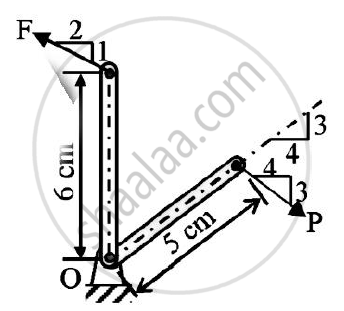Concept: Resultant of concurrent forces
Chapter:  System of Coplanar Forces
 1.2

State Lami’s theorem.
State the necessary condition for application of Lami’s theorem.

Concept: Condition of equilibrium for parallel forces
Chapter:  Equilibrium of System of Coplanar Forces
 1.3

A homogeneous cylinder 3 m diameter and weighing 400 N is resting on two rough inclined surface’s shown.If the angle of friction is 15o.Find couple C applied to the cylinder that will start it rotating clockwise.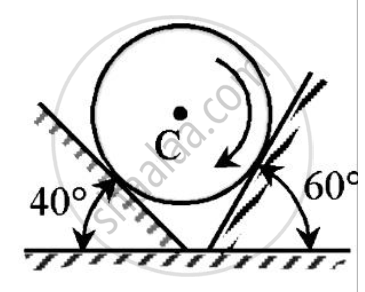Concept: Cone of Friction
Chapter:  Friction
 1.4

From (v-t) diagram find (1) Distance travelled in 10 second.
(2) Total distance travelled in 50 second.
(3) Retardation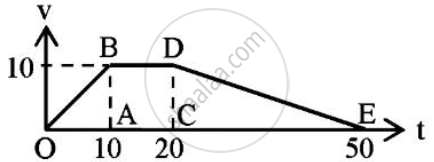Concept: Motion Curves (a-t, v-t, s-t curves)
Chapter:  Kinematics of Particle
 1.5

Blocks P1 and P2 are connected by inextensible string.Find velocity of block P1,if it falls by 0.6 m starting from rest.
The co-efficient of friction is 0.2.The pulley is frictionless.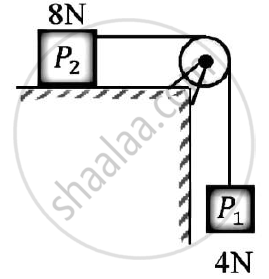Concept: Velocity and Acceleration in Terms of Rectangular Co-ordinate System
Chapter:  Kinematics of Particle
 2
 2.1

Compute the resultant of three forces acting on the plate shown in the figure. Locate it’s intersection with AB and BC.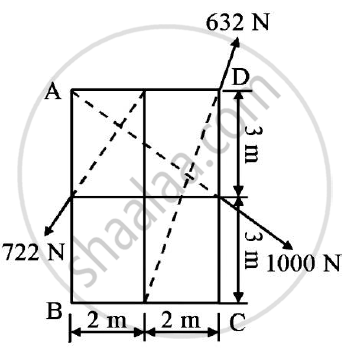Concept: Velocity Diagrams for Bodies in Plane Motion.
Chapter:  Kinematics of Rigid Bodies
 2.2

Two cylinders 1 and 2 are connected by a rigid bar of negligible weight hinged to each cylinder and left to rest in equilibrium in the position shown under the application of force P applied at the center of cylinder 2.
Determine the magnitude of force P.If the weights of the cylinders 1 and 2 are 100N and 50 N respectively.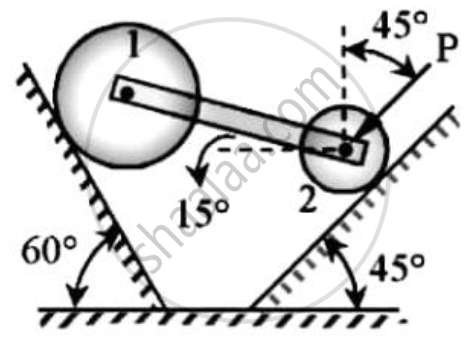Concept: Applications on Equilibrium Mechanisms
Chapter:  Principle of Virtual Work
 2.3

Just before they collide,two disk on a horizontal surface have velocities shown In figure.
Knowing that 90 N disk A rebounds to the left with a velocity of 1.8 m/s.Determine the rebound velocity of the 135 N disk B.Assume the impact is perfectly elastic.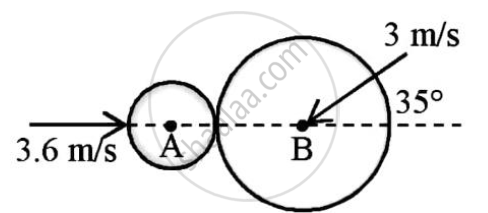Concept: Law of Conservation of Momentum
Chapter: [11.03] Impulse and Momentum
 3
 3.1

Find the centroid of the shaded portion of the plate shown in the figure.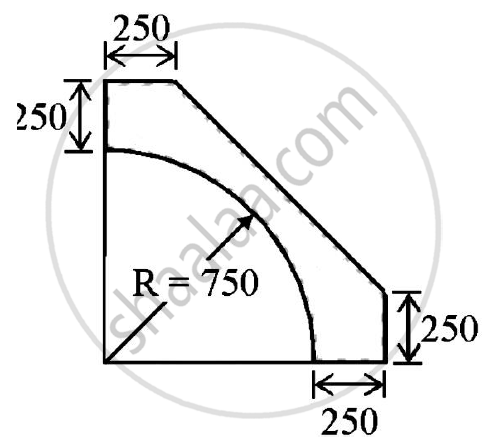Concept: Centroid for Plane Laminas
Chapter:  Center of Gravity and Centroid for Plane Laminas
 3.2

Co-ordinate distance are in m units for the space frame in figure.
There are 3 members AB,AC and AD.There is a force W=10 kN acting at A in a vertically upward direction.
Determine the tension in AB,AC and AD.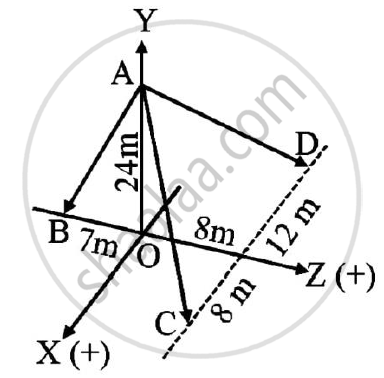Concept: Velocity and Acceleration in Terms of Rectangular Co-ordinate System
Chapter:  Kinematics of Particle
 3.3

A 50 N collar slides without friction along a smooth and which is kept inclined at 60o to the horizontal.
The spring attached to the collar and the support C.The spring is unstretched when the roller is at A(AC is horizontal).
Determine the value of spring constant k given that the collar has a velocity of 2.5 m/s when it has moved 0.5 m along the rod as shown in the figure.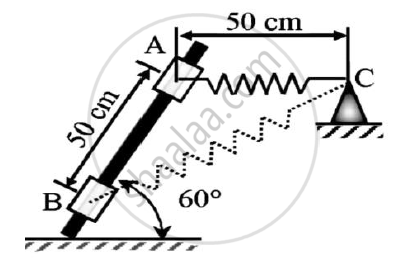Concept: Principle of Work and Energy
Chapter: [11.02] Work and Energy
 4
 4.1

A boom AB is supported as shown in the figure by a cable runs from C over a small smooth pulley at D.
Compute the tension T in cable and reaction at A.Neglect the weight of the boom and size of the pulley.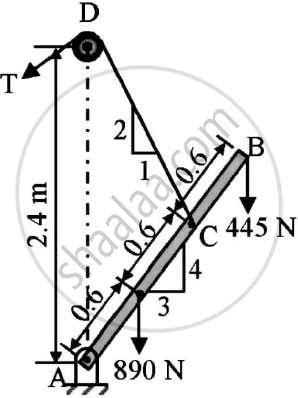Concept: Beams Support
Chapter:  Types of Support
 4.2

The acceleration of the train starting from rest at any instant is given by the expression a=8/(v^2+1) where v is the velocity of train in m/s. Find the velocity of the train when its displacement is 20 m and its displacement when velocity is 64.8 kmph.

Concept: Beams Support
Chapter:  Types of Support
 4.3

Angular velocity of connector BC is 4 r/s in clockwise direction.What is the angular velocities of cranks AB and CD?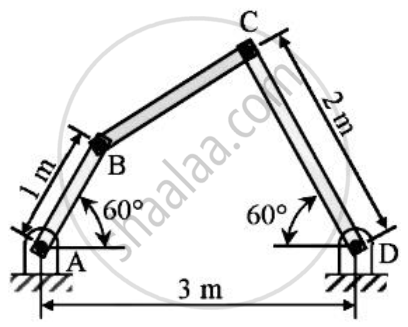Concept: Velocity and Acceleration in Terms of Rectangular Co-ordinate System
Chapter:  Kinematics of Particle
 5
 5.1

In the truss shown in figure,compute the forces in each member.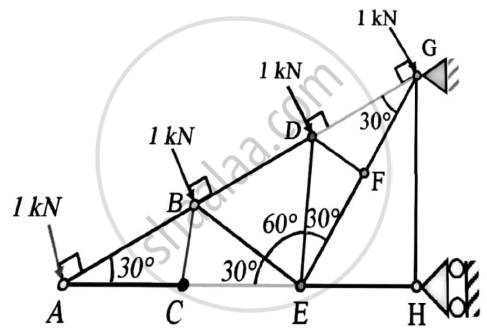Concept: Analysis of Plane Trusses by Using Method of Joints
Chapter:  Analysis of Plane Trusses
 5.2

Determine the speed at which the basket ball at A must be thrown at an angle 30o so that if makes it to the basket at B.
Also find at what speed it passes through the hoop.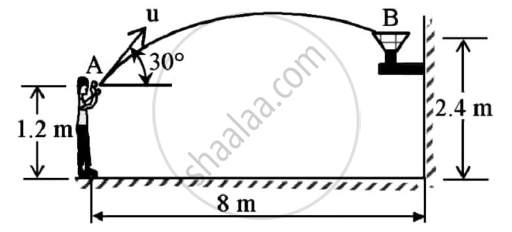Concept: Motion Curves (a-t, v-t, s-t curves)
Chapter:  Kinematics of Particle
 5.3

Figure shows a collar B which moves upwards with constant velocity of 1.5 m/s.At the instant when θ=50o.Determine :
(i)The angular velocity of rod pinned at B and freely resting at A against 25o sloping ground.
(ii)The velocity of end A of the rod.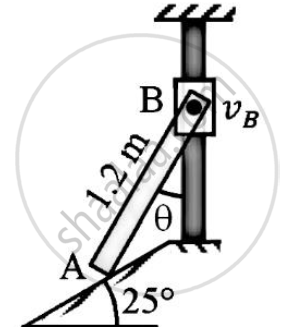Concept: Motion Curves (a-t, v-t, s-t curves)
Chapter:  Kinematics of Particle
 6
 6.1

A force of 140 kN passes through point C (-6,2,2) and goes to point B (6,6,8). Calculate moment of force about origin.

Concept: Moment of force about a point
Chapter:  System of Coplanar Forces
 6.2

Refer to figure.If the co-efficient of friction is 0.60 for all contact surfaces and θ = 30o,what force P applied to the block B acting down and parallel to the incline will start motion and what will be the tension in the cord parallel to inclined plane attached to A.
Take WA=120 N and WB=200 N.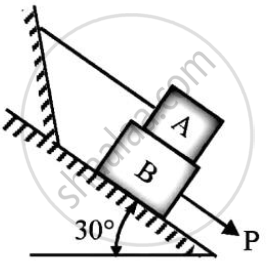Concept: Condition of equilibrium for concurrent forces
Chapter:  Equilibrium of System of Coplanar Forces
 6.3

Determine the required stiffness k so that the uniform 7 kg bar AC is in equilibrium when θ=30o.
Due to the collar guide at B the spring remains vertical and is unstretched when θ = 0o.Use principle of virtual work.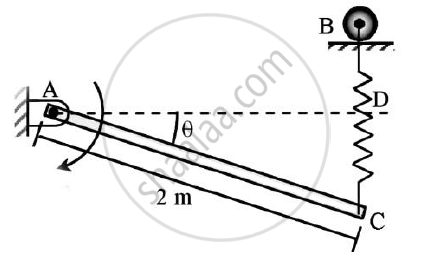Concept: Applications on Equilibrium Mechanisms
Chapter:  Principle of Virtual Work
 6.4

The system in figure is initially at rest.
Neglecting friction determine the force P required if the velocity of the collar is 5 m/s after 2 sec and corresponding tension in the cable.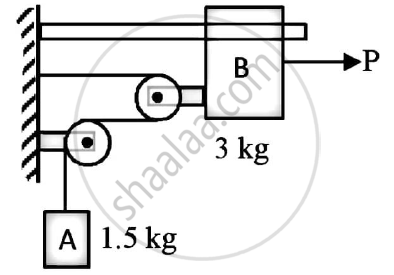Concept: Introduction to Laws of Friction
Chapter:  Friction

#### Request Question Paper

If you dont find a question paper, kindly write to us

View All Requests

#### Submit Question Paper

Help us maintain new question papers on Shaalaa.com, so we can continue to help students

only jpg, png and pdf files

## University of Mumbai previous year question papers Semester 1 (FE First Year) Engineering Mechanics with solutions 2016 - 2017

University of Mumbai Semester 1 (FE First Year) Engineering Mechanics question paper solution is key to score more marks in final exams. Students who have used our past year paper solution have significantly improved in speed and boosted their confidence to solve any question in the examination. Our University of Mumbai Semester 1 (FE First Year) Engineering Mechanics question paper 2017 serve as a catalyst to prepare for your Engineering Mechanics board examination.
Previous year Question paper for University of Mumbai Semester 1 (FE First Year) Engineering Mechanics-2017 is solved by experts. Solved question papers gives you the chance to check yourself after your mock test.
By referring the question paper Solutions for Engineering Mechanics, you can scale your preparation level and work on your weak areas. It will also help the candidates in developing the time-management skills. Practice makes perfect, and there is no better way to practice than to attempt previous year question paper solutions of University of Mumbai Semester 1 (FE First Year).

How University of Mumbai Semester 1 (FE First Year) Question Paper solutions Help Students ?
• Question paper solutions for Engineering Mechanics will helps students to prepare for exam.
• Question paper with answer will boost students confidence in exam time and also give you an idea About the important questions and topics to be prepared for the board exam.
• For finding solution of question papers no need to refer so multiple sources like textbook or guides.